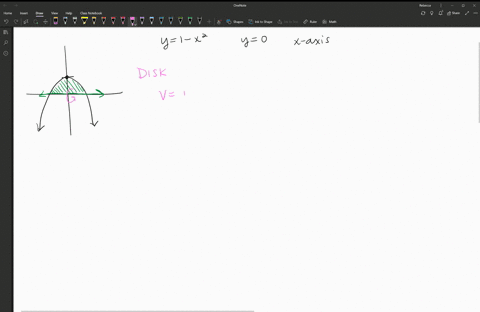Enroll in one of our FREE online STEM summer camps. Space is limited so join now!View Summer Courses### Find the volume of the solid obtained by rotating…

03:01Oklahoma Baptist University

Need more help? Fill out this quick form to get professional live tutoring.

Get live tutoring
Problem 1

Find the volume of the solid obtained by rotating the region bounded by the given curves about the specified line. Sketch the region, the solid, and a typical disk or washer.
$$y=2-\frac{1}{2} x, y=0, x=1, x=2 ; \text { about the } x-axis$$

19 $\mathrm{pi} / 12$

## Discussion

You must be signed in to discuss.

## Video Transcript

do you find the volume of the solid that is rotated of the region, bounded by why equals two minus 1/2 X y equals zero X equals one X equals two and we're going around the x axis. So first of all, let's consider that linear function to minus 1/2 X. This has I intercept of two and then a slope of negative 1/2 so down 1/2 down, 1/2 would make this line. It's about a straight. I could draw it and then we're also looking at y equals zero. So that's gonna be your X axis. We're looking at X is wine, and we're looking at X is too. So the region that's bounded by that linear function X is one excess to And why zeros this part of shaded in green Now for rotating it around the X axis, Then I always want to ask myself, Is there any volume there any space there that needs to be cut out where that's missing? And the answer here is know, as we rotate this, it's gonna be a totally solid figure. I know this because every part of that shaded region and green is coming right up against the exact access. So because it's that solid figure, I know for sure it's a disk method. So for the disk method for volume, we have high R squared and we want to look for the ex boundaries. We want to have it in terms of X with our in a girl because we're going around the X axis. Thankfully, we're given the limits for X. The smallest X value is one the greatest Xlu is, too. That's what's bounding this volume. So I'm gonna put one into on my integral and then to fill in our you're thinking of the Radius. So if I'm looking at my figure, the radius is how far I travel from the center. So where I'm spinning Teoh, you know that that edge of the shape and that are here is determined by going from the linear function to minus 1/2 X down to the axis. So I always like to think of if I'm in terms of X is I like to think of it as upper minus lower. The lower function here, though, is just zero. Because it's the Axis, it's helpful to think upper minus lower for if I have shifted this somewhere else into the plane, so we're gonna fill into minus 1/2 X as that are term here. So then we want to calculate this in a rural. It's pretty easy to go through by hand. If you would just expand that by no meal. So if you just multiply and then you can just do each term by term, so multiplying that out and then going term by term Or if you have a calculation program, you can find this integral total. The integral here is 19/12 so I wouldn't leave my answer exact and call it 19 pi over 12.